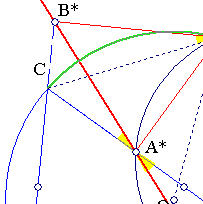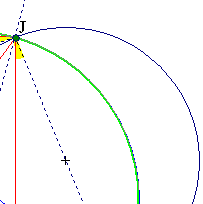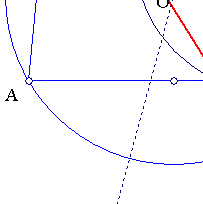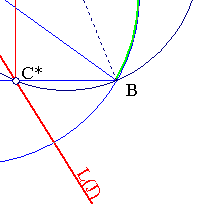##1. Wallace-Simson Line

Project a point J of the circumcircle of triangle ABC on the sides of the triangle. The three projections A*, B*, C* lie on a line L(J), called the Wallace-Simson line of ABC with respect to J.The proof results by an angle chasing argument indicated above. The two marked angles at J are equal because of the cyclic quadrilateral ABJC.

##2. Particular positions

For various positions of J on the circumcircle result particular positions of the corresponding Wallace-Simson line:

 J coincides with a vertex of the triangle. Then L(J) extends the corresponding altitude of the triangle.
 J is the diametral of vertex A. Then L(J) becomes identical with side-line BC of the triangle.
 J is the middle of the arc opposite to A. Then L(J) meets line OJ exactly at the middle of side BC and is parallel to the exterior bisector of angle A.
 J is the middle of the arc containing A. Then L(J) meets line OJ exactly at the middle of side BC and is parallel to the interior bisector of angle A.
 J is the intersection of the altitude from A with the circumcircle. Then L(J) passes through the foot of this altitude and is parallel to the circumcircle-tangent at A.
 L(J) and OJ are parallel <==> L(J) passes through the center of the Euler circle of the triangle.

The proofs follow easily from the discussion in SimsonProperty.html , on the inverse problem of finding a point on the circumcircle whose Simson line is parallel to a given direction.
See SteinerLine.html for the discussion on a line parallel to the Simson line and passing through the orthocenter.

##3. Barycentric coordinates

Conforming to Conway's notation denote by Sw the quantity Scot(w), where S is twice the area of the triangle ABC. In barycentric coordinates the circumcircle is described by the equation:
a2/x + b2/y + c2/z = 0.

##3. Related subjects

 Files ThreeDiameters.html and SimsonRelated.html contain easy exercises relative to this important line.
 File SimsonProperty2.html discusses the relation of the central angle of two points P,Q on the circumcircle with the angle of the corresponding Simson lines L(P), L(Q).
 File SimsonDiametral.html examines the Simson lines of diametral points on the circumcircle.
 File SimsonGeneral.html discusses a generalization of these lines in which the point on the circumference is not projected orthogonally on the sides of the triangle but along an arbitrary (fixed) angle phi.
Problem Characterize the positions J for which the Simson line L(J) passes through the circumcenter.

##4. References

 Lalesco, T. La Geometrie du Triangle. Paris, Jacques Gabay, 1987, pp 8-10.
 Honsberger, R. Episodes in Nineteenth and Twentieth Century Euclidean Geometry. Washington DC, Math. Assoc. Ammer., 1995, p 82.
 Yiu, P. GeometryNotes020402 (pdf). http://www.math.fau.edu/yiu/GeometryNotes020402.pdf, pp 65-67.